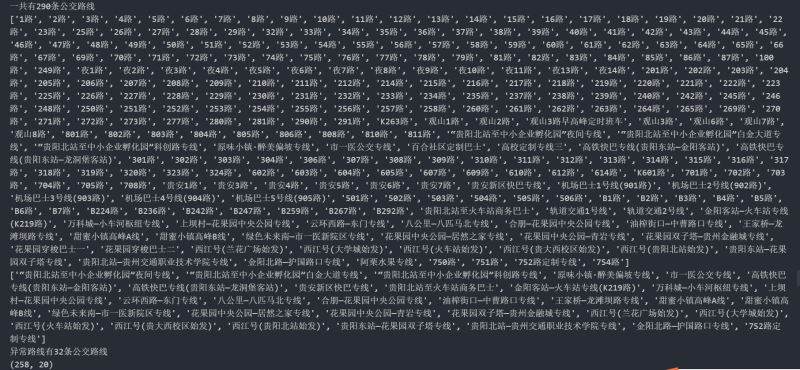﻿ 利用python爬取城市公交站点_python_脚本之家
python# 利用python爬取城市公交站点

## 页面分析

https://guiyang.8684.cn/line1## 爬虫

```import requests
import json
from bs4 import BeautifulSoup
import pandas as pd
​
​
class bus_stop:
## 定义一个类，用来获取每趟公交的站点名称和经纬度
def __init__(self):
self.url = 'https://guiyang.8684.cn/line{}'
self.starnum = []
for start_num in range(1, 17):
self.starnum.append(start_num)
## 调用高德api获取公交线路的经纬度
### 这个key大家可以自己去申请
def get_location(self, line):
url_api = 'https://restapi.amap.com/v3/bus/linename?s=rsv3&extensions=all&key=559bdffe35eec8c8f4dae959451d705c&output=json&city=贵阳&offset=2&keywords={}&platform=JS'.format(
line)
res = requests.get(url_api).text
# print(res) 可以用于检验传回的信息里面是否有自己需要的数据
dicts = rt['buslines']
# 返回df对象
df = pd.DataFrame.from_dict([dicts])
return df
## 获取每趟公交的站点名称
def get_line(self):
for start in self.starnum:
start = str(start)
# 构造url
url = self.url.format(start)
res = requests.request(
soup = BeautifulSoup(res.text, "lxml")
div = soup.find('div', class_='list clearfix')
lists = div.find_all('a')
for item in lists:
line = item.text  # 获取a标签下的公交线路
lines.append(line)
return lines
​
​
if __name__ == '__main__':
bus_stop = bus_stop()
stop_df = pd.DataFrame([])
lines = []
bus_stop.get_line()
# 输出路线
print('一共有{}条公交路线'.format(len(lines)))
print(lines)
# 异常处理
error_lines = []
for line in lines:
try:
df = bus_stop.get_location(line)
stop_df = pd.concat([stop_df, df], axis=0)
except:
error_lines.append(line)

# 输出异常的路线
print('异常路线有{}条公交路线'.format(len(error_lines)))
print(error_lines)

# 输出文件大小
print(stop_df.shape)
stop_df.to_csv('bus_stop.csv', encoding='gbk', index=False)
```## 数据清洗### Excel PQ 数据清洗### python数据清洗

```## 我们需要处理的busstops列和ID列
data = stop_df[['id','busstops']]
``````## 字典或者列表分列
df_pol = data.copy()
### 设置索引列
df_pol.set_index('id',inplace=True)
``````## 逆透视
### 释放索引
df_pol.reset_index(inplace=True)
### 逆透视操作
df_pol_ps = df_pol.melt(id_vars=['id'], value_name='busstops')
``````## 删除空行
df_pol_ps.dropna(inplace=True,axis=0)
df_pol_ps.shape ``````## 分列
### 设置line_id
df_parse['line_id'] = df_pol_ps['id']
df_parse = df_pol_ps['busstops'].apply(pd.Series)
df_parse
``````## 写入文件
df_parse.to_excel('贵阳市公交站点分布.xlsx', index=False)</pre> ```

## QGIS坐标纠偏

QGIS基础操作，我就不说了，顺便说一下QGIS对csv格式支持较好，我推荐我们导入QGIS的文件为csv格式的文件。

### 导入csv文件### 坐标纠偏